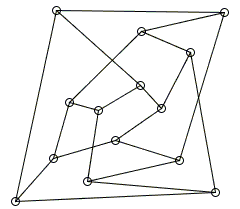# Crossing Number of a Graph

A graph which is essentially an algebraic structure of nodes and edges is commonly presented geometrically by diagrams depicting nodes as dots and edges as curves or straight line segments that connect the dots. Such graphic representations are far from being unique. Below you can see several such avatars of the Petersen graph [Balakrishnan, iv]:An obvious fact about these diagrams is that sometimes the edges cross at the points that do not belong to the graph. In other words, in diagramatic incarnations, the edges may occasionally meet at the points that are not the nodes of the graph. For planar graphs, there is always a representation that avoids such crossings at all. But not all graphs are planar. And for the latter kind, an important characteritics is their crossing number, the minimal number of edge crossings among all possible diagramatic representations of the graph in a plane. Note that, for the sake of the crossings count, avatars like the second in the upper row above are not allowed. What disqualifies it is the presence of a crossing where more than two edges meet. However, it is always possible either by edge distortion or by shifting the nodes to change the diagram to a permissible one (the right most in the upper row). The crossing number of a graph is often denoted as k or cr.

Among the six incarnations of the Petersen graph, the middle one in the bottom row exhibits just 2 crossings, fewer than any other in the collection. In fact, 2 is the crossing number of the Petersen graph. Try as you may, it is impossible to diagram the Petersen graph with one or zero crossings. The fact begs a proof. In the proof below, I'll use an argument suggested by Nathan Bowler in one of the online discussions at the CTK Exchange.

Necessary for the proof is the notion of girth. The girth of a graph is the length of the shortest cycle the graph contains. (Here I assume that the graph does not have parallel edges, i.e. edges of multiplicity higher than 1, nor the loops.) I shall use the symbol c to denote the girth. Always c ≥ 3. For the Petersen graph, c = 5. (Looking at the leftmost avitar in the upper row above, the outer pentagon and the inner star are 5-cycles. Other cycles are bound to contain an even number of "bridges". But if two bridges are adjacent at one end they are at least two edges apart at the other.)

For every avatar, let's consider a subgraph obtained by removing one of the edges at every crossing present. This operation is not going to increase the graph's girth. Furthermore, the operation leaves a graph with no crossings, i.e., a planar graph. For the planar graphs, we have Euler's polyhedral formula:

f - e + v = 2,

where f, e, and v, are correspondingly the number of faces (countries), edges, and vertices of a graph. For a planar graph with girth c,

fc ≤ 2e.

The two formulas together give

 (1) 2e - ce + cv ≥ 2c.

If E is the original number of edges, then e = E - k and inequality (1) implies that

 (2) k ≥ E - (v - 2)·c/(c - 2).

For the Petersen graph, E = 15, v = 10, c = 5, so that (2) gives

k ≥ 5/3,

or since k is an integer,

k ≥ 2.

Finally, since in one of the above diagram the number of crossings is exactly 2, we conclude that, for the Petersen graph,

k = 2.

Another example of using (2) is served by the Heawood graph:For the graph, c = 6 (this needs a proof), E = 21, v = 14. Substituting these into (2) gives

k ≥ 21 - 12·6/4 = 3.

But it is possible to depict the Heawood graph with just three crossings:implying that its crossing number is exactly 3.

For the prototypical non-planar graphs K3,3 and K5 (2) also proves useful. Indeed, for K3,3, c = 4, E = 9, v = 6, so that k ≥ 1. For K5, c = 3, E = 10, v = 5, so that again k ≥ 1. In this manner, via (2), we get an additional proof of the non-planarity of K3,3 and K5.

### Remarks

1. Since c ≥ 3, (2) implies that for planar graphs

E ≤ 3v - 6.

For K5 we would have, 10 ≤ 9, which again shows that the graph is non-planar.

2. Richard Guy showed (1972) that for complete graphs Kn,

k(Kn) ≤ [n/2][(n-1)/2][(n-2)/2][(n-3)/2]/4,

with equality proven for small n. To the best of my knowledge, his conjecture that the equality always holds is still unproven.

### Reference

1. V. K. Balakrishnan, Graph Theory, Schaum's Outlines, 1997
2. N. Hartsfield, G. Ringel Pearls in Graph Theory: A Comprehensive Introduction, Dover, 1994
3. R. J. Trudeau, Introduction to Graph Theory, Dover, NY, 1993.